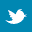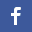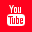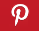Completely Solved C, C++ Programs Assignment.Quick Search Database Videos Tutorials Ebooks Forums FAQ Aboutus Household Industrial Manufacturing Service Shopping Transportation### C program to implement Gauss-Seidel method to solve a system of equations.

 Filed Under: C Assignments

Program Statement :
Write a C program to implement Gauss-Seidel method to solve a system of equations.

Theory :
In numerical linear algebra, the Gauss–Seidel method, is an iterative method used to solve a linear system of equations.
To illustrate this method, we rewrite the system of equations
a11x1 + a12x2+ a13x3 +...+ a1nxn = b1
a21x1 + a22x2 + a23x3 +...+ a2nxn = b2
a31x1 + a32x2 + a33x3 +...+ a3nxn = b3
. . . . . .
. . . . . .
. . . . . .
an1x1 + an2x2 + an3x3 +...+ annxn = bn
in the form,
n n
xi = 1/aii [ bi - ∑ aijxj(k+1) - ∑ aijxj(k+1) ], (i = 1, 2,..., n)
j=1 j=1
ji
provided that aii != 0, (i = 1, 2,..., n).
To solve the above equation, suppose that xi(0), (i = 1, 2,..., n), be the initial approximations (usually xi(0) are taken to be zero) of the solutions of the system of equations. We substitute these initial values on the right hand side of the first equation of the above equation, i.e., when i = 1 and we get the first approximation of x1 as
x1(1) = 1/a11 [ b1 - a12x2(0) - a13x3(0) ... a1nxn(0) ].
In the second equation, i.e., for i = 2, we substitute the improved value x1(1) and initial values x3(0), x4(0),..., xn(0) and obtain the first approximation of x2 as
x2(1) = 1/a22 [ b2 - a21x1(1) - a23x2(0) - ... - a2nxn(0) ].
We then substitute in the third equation, i.e., for i = 3, we substitute the improved values x1(1), x2(1) and the initial values x4(0), x5(0),..., xn(0) and obtain the first approximation of x3 as
x3(1) = 1/a33 [ b3 - a31x1(1) - a32x2(1) - ... - a3nxn(0) ].
Proceeding in this way, the first approximation of xn is given by
xn(1) = 1/ann [ bn - an1x1(1) - an2x2(1) - ... - an n-1xn-1(1) ].

Thus at the end of the first stage of iteration, we get the first approximation x1(1), x2(1),..., xn(1) to the solutions x1, x2,..., xn.
Now if xik, (k = 0,1, 2,...), be the k-th approximation to the solutions xi, (i = 1, 2,..., n), then the (k+1)-th approximation xi(k+1) of xi, (i = 1, 2,..., n), are given by
x1(k+1) = 1/a11 [ b1 - a12x2(k) - a13x3(k) ... a1nxn(k) ].
x2(k+1) = 1/a22 [ b2 – a21x1(k+1) - a23x3(k) ... a2nxn(k) ].
... ... ... ... ...
xn(k+1) = 1/ann [ bn – an1x1(k+1) - an2x2(k) ... an n-1xn-1(k+1) ].
or, in compact form, we have
i-1 n
xi(k+1) = 1/aii [ bi – ∑ aijxj(k+1) - ∑ aijxj(k) ], (i = 1, 2,..., n),
j=1 j=i+1
The process is continued until we get the solutions x1, x2,..., xn with sufficient degree of accuracy.

Algorithm :
Step1 : Start the program.
Step2 : Read the augmented matrix
[aij], i = 1 to n; j = 1 to (n+1)
Step3 : Enter the initial approximation xi = 0, i = 1 to n.
Step4 : For i = 1(1)n.
Step5 : Set s = ai n+1
Step6 : For j = 1(1)n.
Step7 : If i != j, set s = s - aijxj
Step8 : Else, next j
Step9 : xi = s/aii
Step10 : Print the value of xi (i = 1, 2,..., n).
Step11 : Stop the algorithm.

Program listing :
/* C program to implement Gauss-Seidel Iteration Method */
#include<stdio.h>
#define max 50
#define e 0.00001/*e is the prescribed accuracy*/
main()
{
float a[max][max],x[max];
int n,i,j;
int gauss_seidel(float [][max],float [],int);
void printmat(float [][max],int);
printf("Enter the order of the matrix(n x n) : ");
scanf("%d",&n);
if(n>0)
{
/*The user enters the augmented matrix*/
printf("nEnter the augmented matrix row wise :-n");
for(i=0;i<n;i++)
{
printf("nEnter row %d :-n",i+1);
for(j=0;j<(n+1);j++)
{
printf("Enter element %d: ",j+1);
scanf("%f",&a[i][j]);
}
}
printf("nThe augmented matrix entered is :-n");
printmat(a,n);
if(gauss_seidel(a,x,n))/*The gauss_seidel function return true value */
{
printf("nThe solutions of the given equations are->n");
for(i=0;i<n;i++)
printf("ntx[%d]=%10.2fn",i+1,x[i]);
}
}
else
printf("nNo solution.");
}
/*Functions implemnts Gauss-Seidel on a system of equations*/
int gauss_seidel(float a[][max],float x[],int n)
{
int i,j,p=1;
float x1[max];
float s;
int diagonal_dominant(float [][max],int);
int converge(float [max],float [max],int);
if(!diagonal_dominant(a,n))/*Checking the diagonal dominant condition*/
{
printf("nThe system is not diagonally dominant.");
return 0;/*The solution cannot be obtained if system is not diagonal-dominant*/
}
/*Taking the initial approximation as zero*/
for(i=0;i<n;i++)
{
x1[i]=0;
x[i]=0;
}
do
{
printf("nn");
printf("Iteration %d :",p);
p++;
for(i=0;i<n;i++)
{
s=0;
for(j=0;j<n;j++)
{
if(i!=j)
s+=a[i][j]*x[j];
}
x[i]=(a[i][n]-s)/a[i][i];
printf("nx[%d]=%10.6f",i+1,x1[i]);
}
/*If the present result converges to the last result then we stop */
if(converge(x,x1,n))
break;
for(i=0;i<n;i++)
x1[i]=x[i];
}while(1);
printf("n");
return 1;
}
float absolute(float x,float y)/*Returns the absolute value*/
{
float t=x-y;
if(t>0.0)
return t;
return (-1.0)*t;
}
/*Function checking diagonal-dominance*/
int diagonal_dominant(float a[][max],int n)
{
int i,j;
float s;
for(i=0;i<n;i++)
{
s=0;
for(j=0;j<n;j++)
{
if(i!=j)
s+=absolute(a[i][j],0.0);
}
if(absolute(a[i][i],0.0)<s)
return 0;
}
return 1;
}
/*Function checking convergence*/
int converge(float x[max],float x1[max],int n)
{
int i;
for(i=0;i<n;i++)
{
if(absolute(x[i],x1[i])>e)
return 0;
}
return 1;
}
/*Printing the augmmented matix*/
void printmat(float b[][max],int n)
{
int i,j;
for(i=0;i<n;i++)
{
for(j=0;j<(n+1);j++)
{
if(j==n)
printf(" |");
printf(" %10.6f ",b[i][j]);
}
printf("n");
}
}

Output :
Enter the order of the matrix(n x n) : 3
Enter the augmented matrix row wise :-

Enter row 1 :-
Enter element 1: 5
Enter element 2: 2
Enter element 3: 1
Enter element 4: 12

Enter row 2 :-
Enter element 1: 1
Enter element 2: 4
Enter element 3: 2
Enter element 4: 15

Enter row 3 :-
Enter element 1: 1
Enter element 2: 2
Enter element 3: 5
Enter element 4: 20

The augmented matrix entered is :-
5.000000 2.000000 1.000000 | 12.000000
1.000000 4.000000 2.000000 | 15.000000
1.000000 2.000000 5.000000 | 20.000000

Iteration 1 :
x= 0.000000
x= 0.000000
x= 0.000000

Iteration 2 :
x= 2.400000
x= 3.150000
x= 2.260000

Iteration 3 :
x= 0.688000
x= 2.448000
x= 2.883200

Iteration 4 :
x= 0.844160
x= 2.097360
x= 2.992224

Iteration 5 :
x= 0.962611
x= 2.013235
x= 3.002184

Iteration 6 :
x= 0.994269
x= 2.000341
x= 3.001010

Iteration 7 :
x= 0.999662
x= 1.999580
x= 3.000236

Iteration 8 :
x= 1.000121
x= 1.999852
x= 3.000035

Iteration 9 :
x= 1.000052
x= 1.999969
x= 3.000002

Iteration 10 :
x= 1.000012
x= 1.999996
x= 2.999999

Iteration 11 :
x= 1.000002
x= 2.000000
x= 3.000000

The solutions of the given equations are->

x= 1.00

x= 2.00

x= 3.00

Discussions :
 This method is an improvement of the Gauss – Jacobi method in the sense that the improved values of xi are used here in each iteration instead of the values of the previous iteration and hence the method of successive displacement.
 Though this method can be applied to any matrix with non-zero elements on the diagonals, convergence is only guaranteed if the matrix is either diagonally dominant, or symmetric and positive definite. It may be noted that the strictly diagonally dominant condition may not be necessary in some problems for the convergence of iteration.
 The order of convergence of iteration in Gauss-Seidel method is one.
 Since the improved values of the unknowns are used at each stage of iteration, the rate of convergence is faster (roughly twice) than that of Gauss-Jacobi method.
 The computation of xi(k+1) uses only the elements of x(k+1) that have already been computed, and only the elements of x(k) that have yet to be advanced to iteration k+1. This means that, unlike the Jacobi method, only one storage vector is required as elements can be overwritten as they are computed, which can be advantageous for very large problems.
 However, unlike the Jacobi method, the computations for each element cannot be done in parallel. Furthermore, the values at each iteration are dependent on the order of the original equations.
 The convergence properties of the Gauss-Seidel method are dependent on the matrix A. Namely, the procedure is known to converge if either :
1. A is symmetric positive-definite, or
2. A is strictly or irreducibly diagonally dominant.
The Gauss–Seidel method sometimes converges even if these conditions are not satisfied.
 It, therefore, follows that the sequence {xi(k)} generated from the following equation.
i-1 n
xi(k+1) = 1/aii [ bi – ∑ aijxj(k+1) - ∑ aijxj(k) ], (i = 1, 2,..., n),
j=1 j=i+1
converges to the solution {xi*} if 0 < ∝ < 1, i.e., the iteration converges if n |aii| > ∑ |aij|, (i = 1, 2,..., n).
j=1
j!=iBack to main directory:Software Practical